# AP Board 9th Class Maths Solutions Chapter 14 Probability InText Questions

AP State Syllabus AP Board 9th Class Maths Solutions Chapter 14 Probability InText Questions and Answers.

## AP State Syllabus 9th Class Maths Solutions 14th Lesson Probability InText QuestionsDo This

Question 1.
Observe the table given in the previous page (Textbook Page No. 293) and give some other example for each term. [Page No. 294]
Solution:
Certain : Independence day on 15th Aug.
More likely : When a die is thrown, the chance of getting a number less than or equal to 5. Equally likely : When a coin is tossed, getting a head.
Less likely : When a die is thrown, the chance of getting neither prime nor composite. Impossible : When a die is thrown, getting a negative number.

Question 2.
Classify the following statements into the categories less likely, equally . likely, more likely. [Page No. 294]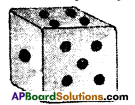a) Rolling a die and getting a number 5 on the top face.
b) Getting a cold wave in your village in the month of November.
c) India winning the next soccer (foot ball) world cup.
d) Getting a tail or head when a coin is tossed.
e) You buy a lottery ticket and win the jackpot.
Solution:
a) less likely
b) more likely
c) less likely
d) equally likely
e) more likelyTry These

Question 1.
If you try to start a scooter, what are the possible outcomes ? [Page No. 295]
Solution:
[Starts], [Doesn’t start]

Question 2.
When you roll a die, what are the six possible outcomes ? [Page No. 295]
Solution:
1, 2, 3, 4, 5 and 6.

Question 3.
When you spin the wheel shown, what are the possible outcomes ? (Outcomes here means the possible sector where the pointer stops) [Page No.295]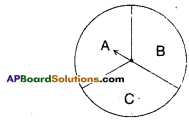Solution:
A, B and C.

Question 4.
You have ajar with five identical balls of different colours. [White, Red, Blue, Grey and Yellow] and you have to pick up (draw) a ball without looking at it. List the possible outcomes you get. [Page No. 295]Solution:
White ball, Red ball, Blue ball. Grey ball and Yellow ball.Think, Discuss and Write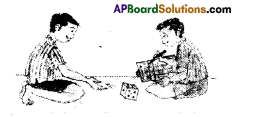In rolling a die [Page No. 295]

Question
Does the first player have a greater chance of getting a six on the top face ?
Solution:
No. The chance of getting 6 on the top face is independent of the turn of the player.

Question
Would the player who played after him have a lesser chance of getting a six on the top face?
Solution:
No.Question
Suppose the second player got a six on the top face. Does it mean that the third player would not have a chance of getting a six on the top face ?
Solution:
No. The third player may or may not get six on the top face. It is indepen¬dent of 2nd player’s outcome.

Do This

Question
Toss a coin for number of times as shown in the table. And record your findings in the table [Page No. 296]
Solution: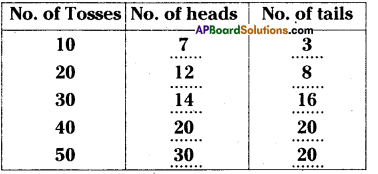Question
What hapens if you increase the num- her of tosses more and more.
Solution:
If you increase the number of tosses more and more they are equally likely chances to get a head or a tail.
Note : This could also be done by the students with a die, roll it for large number of times and observe!Do This

If three coins are tossed simulta¬neously : [Page No. 299]

a) Write all possible outcomes.
Solution:
HHH, HHT, HTH, THH, HTT, THT, TTH, TTT, total 8 outcomes.

b) Number of possible outcomes.
Solution:
8

c) Find the probability of getting at least one head, (getting one or more than one head)
Solution:
P = $$\frac{\text { favourable outcomes }}{\text { total outcomes }}=\frac{7}{8}$$

d) Find the probability of getting at most two heads, (getting two or less than two heads)
Solution:
P = $$\frac{\text { favourable outcomes }}{\text { total outcomes }}=\frac{7}{8}$$e) Find the probability of getting no tails.
Solution:
P = $$\frac{\text { favourable outcomes }}{\text { total outcomes }}=\frac{1}{8}$$

Try This

Find the probability of each event when a die is rolled once. [Page No. 300]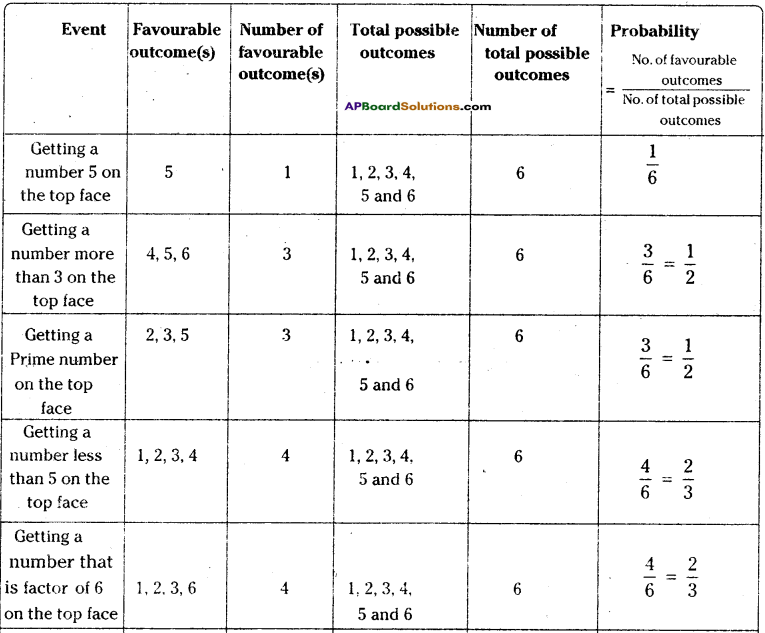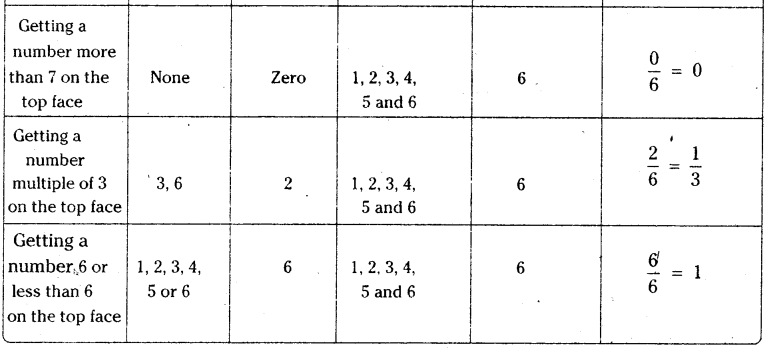Try These

From the figure given below [Page No. 306]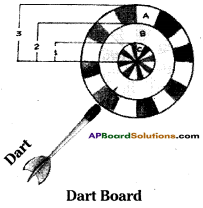Question 1.
Find the probability of the dart hit¬ting the board in the circular region B. (i.e., ring B)
Solution:
Area of innermost ‘C’ circle = πr2
= π x 12 = π sq. units.
Area of the middle ‘B’ circle
= π (22 – 12) = π (4 – 1) = 3π sq.units.
Area of the outermost ’A’ circle
= π (32 – 22) = π (9 – 4) = 5π sq.units.
Probability of hitting the circle B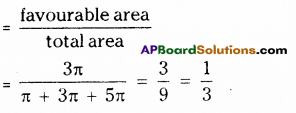Question 2.
Without calculating, write the percent¬age of probability of the dart hitting the board in circular region ‘C’ (Le., ring C).
Solution:
$$\frac{1}{9}$$ x 100% = 11$$\frac{1}{9}$$